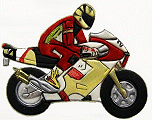Go to content
If v represents the velocity of an object at time t then s = \displaystyle \int v \, dt will represent its position.## Software/Applets used on this pageThis page uses jsMath
You can get a better display of the maths by downloading special TeX fonts from jsMath. In the meantime, we will do the best we can with the fonts you have, but it may not be pretty and some equations may not be rendered correctly.

## Glossary

### union

The union of two sets A and B is the set containing all the elements of A and B.

### velocity

the rate of change of displacement. It is a vector quantity with magnitude and direction.

Full Glossary List

## This question appears in the following syllabi:

SyllabusModuleSectionTopicExam Year
AP Calculus AB (USA)4KinematicsUse of calculus-
AP Calculus BC (USA)4KinematicsUse of calculus-
AQA A-Level (UK - Pre-2017)M2KinematicsUse of calculus-
AQA A2 Further Maths 2017MechanicsExtraMotion Calculus-
AQA AS Maths 2017MechanicsKinematics and UnitsUsing Calculus-
AQA AS/A2 Further Maths 2017MechanicsExtraMotion Calculus-
AQA AS/A2 Maths 2017MechanicsKinematics and UnitsUsing Calculus-
CCEA A-Level (NI)M1KinematicsUse of calculus-
CIE A-Level (UK)M1KinematicsUse of calculus-
Edexcel A-Level (UK - Pre-2017)M2KinematicsUse of calculus-
Edexcel AS Further Maths 2017Further Mechanics 2KinematicsUsing Calculus-
Edexcel AS Maths 2017MechanicsVariable Acceleration MotionUsing Calculus-
Edexcel AS/A2 Further Maths 2017Further Mechanics 2KinematicsUsing Calculus-
Edexcel AS/A2 Maths 2017MechanicsVariable Acceleration MotionUsing Calculus-
I.B. Higher Level6KinematicsUse of calculus-
I.B. Standard Level6KinematicsUse of calculus-
Methods (UK)M9KinematicsUse of calculus-
OCR A-Level (UK - Pre-2017)M1KinematicsUse of calculus-
OCR A2 Further Maths 2017MechanicsFurther Dynamics and KinematicsUsing Calculus-
OCR AS Maths 2017MechanicsWorking with MotionUsing Calculus-
OCR MEI A2 Further Maths 2017Mechanics BVectors and Variable ForcesUsing Calculus-
OCR MEI AS Maths 2017MechanicsWorking with MotionUsing Calculus-
OCR-MEI A-Level (UK - Pre-2017)M1KinematicsUse of calculus-
Pre-U A-Level (UK)MechKinematicsUse of calculus-
Scottish Advanced HighersM1KinematicsUse of calculus-
Scottish (Highers + Advanced)AM1KinematicsUse of calculus-
Universal (all site questions)KKinematicsUse of calculus-
WJEC A-Level (Wales)M2KinematicsUse of calculus-